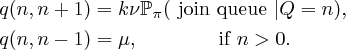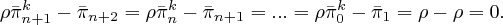# Power-of-k Choices

We consider a model of a large number of single server queues. When a job arrives, we assume it chooses between$k$ queues. The job then chooses to join the shortest of these queues. We show that such choice can dramatically reduce queue sizes.

• Consider, in continuous-time, a single server queue with service rate$\mu$. Jobs, which have independent exponentially distributed mean 1 size. We assume arriving jobs observe the queue as an independent Poisson process of rate$k\nu$.
• We assume$\nu<\mu$ – this is sufficient for the queue to be stationary – and we let$\pi_n$ be the stationary probability of there being$n$ customers at the queue. As is conventional, we define$\rho=\nu/\mu$.
• We assume that when a job observes our queue it also observes$k-1$ other queues. These queues are independent with distribution the same as$\pi_n$. The job joins our original queue if its length is smaller than these other independent queues. If the queue lengths are equal then the job chooses amongst those queues at random.

Here we implicitly consider a model with large number of identical single server queues. A formal limit which increases the number of queues is called a mean field limit. We do not consider such a formal justification. When$k$ of these queues are chosen uniformly at random, we assume that these queues have interacted so infrequently that they are statistically independent. Thus we may study one queue in isolation and, at each observation, consider it to be interacting with$k-1$ queues which are each independent and equal in distribution. We then search for a stationary distribution which is fixed under interaction with$k-1$ independent instance of this stationary distribution. This notion of solving for distribution by interacting with independent instance of itself is sometimes referred to as the cavity method.

Theorem: For a power of$k$ choices queue with$k\geq 2$ and$\rho<1$, the stationary probability the queue has length greater than or equal to$n\in {\mathbb Z}_+$,$\bar{\pi}_n$, is given by• With choice, a single sever M/M/1 has a geometric stationary distribution$\pi_n=\rho^{n}$. Has power of$k$ choices queue has a dramatically smaller queue size.
• A proportion,$\pi_0=1-\rho$, of queues are empty i.e. there is always an empty queue to choose. So, as$k\rightarrow\infty$ all queues are either empty with probability$\pi_0=1-\rho$ or have a single customer with probability$\pi_1=\rho$. So the whole system behaves as an$M/M/\infty$ queue and all resources are pooled together.

Proof: The transition rates of our queueing networks can be expressed asNow, let’s first calculate the probability of a job observing our queue joins the queue given it’s length is$n$.Each term accounts that amongst the$k-1$ queues there are$\kappa-1$ queues of size equal to$n$ and all remaining queues have size greater than$n$, then given this, the probability our single server queue is selected by the job is$1/\kappa$.

Observe the above expression is extremely close to the expansion of$( \bar{\pi}_{n+1} + \pi_n )^k=\bar{\pi}_n^k$. We are missing one term,$\pi_{n+1}^k$, and a multiplicative factor of$k\pi_n$. This observation is the crux of the argument.

Now, using detailed balance, let’s calculate the stationary distribution of this system.Noting$\pi_{n+1}=\bar{\pi}_{n+1}-\bar{\pi}_{n+2}$ and rearranging the above expression, observe that the term$\rho \bar{\pi}^k_n-\bar{\pi}_{n+1}$ is constant over$n$:In the penultimate equality above, we use that$\bar{\pi}(0)=1$ and$\bar{\pi}(1)=\rho$. We know$\bar{\pi}(1)=\rho$ because the long run arrival rate of work,$\nu$, equals the long run service rate of work,$\mu \bar{\pi}_1$. 1 As$\rho \bar{\pi}^k_n-\bar{\pi}_{n+1}=0$ over all$n$, iterating to$\bar{\pi}_0=1$ gives$\square$

1. A queue is observed at rate$k\nu$. By symmetry, the (unconditioned) probability a job joins any of the$k$ observed queues is$1/k$. Thus, the long run arrival rate is$\nu$. In addition, a non-idling queue only processes work when it is non-empty, i.e.$\bar{\pi}_1$, and by assumption a single server queue processes this work at rate$\mu$. Hence for a stable queue the long-run arrival rate of work equals the long-run service rate,$\nu=\mu\bar{\pi}_1$ and so$\bar{\pi}_1=\rho$.